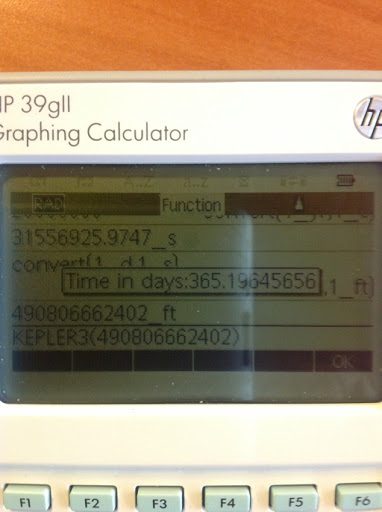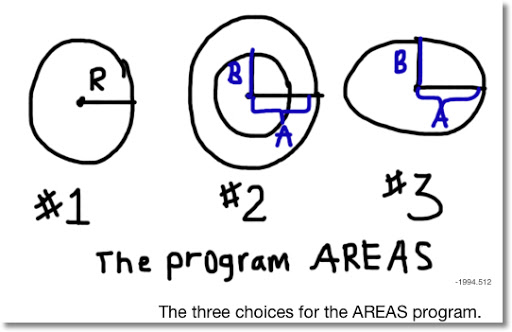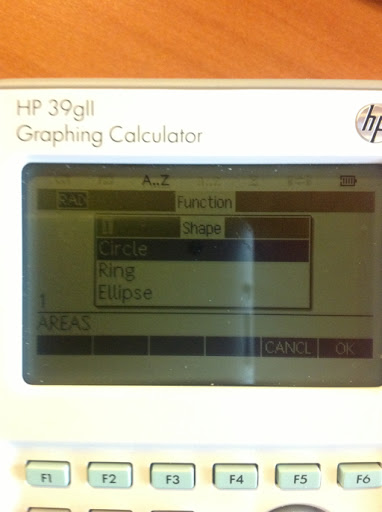## Saturday, March 9, 2013

### HP 39gii Programming Part 2: IF/THEN/ELSE/END, MSGBOX, CHOOSE

1. Comparative Operators
2. Storing and assignments
3. IF-THEN-ELSE-END
4. MSGBOX
5. CHOOSE

1. Comparative Operators

The six comparative operators are:

== Equals (Two equal signs)
≠ Not Equals
> Greater Than
≥ Greater or Equal Than
< Less Than
≤ Less or Equal Than

The result of each comparison is either 1 (the comparison is true) or 0 (the comparison is false).

Keystrokes: [Math] [X,T,θ,N],
[ 1 ] for <
[ 3 ] for ==
[ 4 ] for >
[ 9 ] for ≠
[Vars] for ≤
[Math] for ≥

2. Storing and Assignment

There are two ways to store objects:

One is by the STO> command, which usually lives in [ F1 ].

Example: 11>A

The other is by the construct := (colon then equals sign). Keystrokes [ALPHA] [ . ] [SHIFT] [ . ]

Example: A:=11

I am not sure there is any difference between the two storing methods. In this tutorial I will use the latter. (colon followed by an equals sign)

3. IF-THEN-ELSE-END

The structure of IF-THEN-ELSE-END:
IF comparison
THEN commands if the comparison is true;
ELSE commands if the comparison is false;
END;

The ELSE part is optional.

Let's use the IF-THEN-ELSE-END structure in an example. The program ISEVEN, which uses a pass-through argument, tests whether the given number is odd or even.

I usually type the IF-THEN-ELSE-END structure. In the programming edit mode, you can also insert the template with the following keystrokes: [ F6 ] (TEMPLT) [ 2 ] [ 2 ].

The program ISEVEN
EXPORT ISEVEN(N)
BEGIN
N:=INT(N);
IF FRAC(N/2)==0
THEN PRINT(string(N) + " is even.");
ELSE PRINT(string(N) + " is odd.);
END;

Testing:

ISEVEN(25) prints "25 is odd."
ISEVEN(66) prints "66 is even."

4. MSGBOX

MSGBOX is a nice way to display messages in pop-up dialog boxes.

Syntax:
MSGBOX(string of text);

Either type the command or use the following keystroke sequence: [SHIFT] [Math] [ F1 ] [ 5 ] [ 8 ]

Let's demonstrate the use of MSGBOX in the program KEPLER3. KEPLER3 demonstrates Kepler's Third Law. This program in particular tells you how many days an object orbits the Sun of our Solar System. Using U.S. units (I will list SI units too, but the program I demonstrate will use U.S. units)

The mass of the sun is approximately 4.38521 x 10^30 lbs. (1.9891 x 10^30 kg)

The Universal Gravitational constant is about 1.069104 x 10^-9 ft^3/(lb s^2).
(6.67428 x 10^-11 m^3/(kg s^2))

There are 86,400 seconds in a day.

KEPLER3 will also demonstrate the use of local variables. I recommend typing the command LOCAL. This program uses a pass-through argument, average radius.

The Program KEPLER3
EXPORT KEPLER3(R)
BEGIN
LOCAL G,M,T;
G:=1.069104E-9;
M:=4.38521E30;
T:=√((4*π^2*R^3)/(G*M))/86400;
MSGBOX("Time in Days:" + string(T));
END;

Access the "E" in numbers (stands for 10^) by pressing [SHIFT] [X,T,θ,N] (EEX).

Test: If the Earth orbits the Sun in a (near) circular motion maintaining a radius of approximately 490,806,662,402 feet, then approximately how many days does it take the Earth to orbit the sun?

KEPLER3(490806662402) returns in a pop-up box, "Time in Days:365.19645656".

Reasonable estimate don't you think?5. CHOOSE

The syntax of the CHOOSE command is:
CHOOSE(var, "Title string", "Choice1", "Choice2", ...);

The choice number is stored in var.

CHOOSE can be typed or called using the keystroke sequence [SHIFT] [Math] [ F1 ] [ 5 ] [ 1 ].

If the user cancels out of the choose box, then var will have the value of 0. var can either be a global real variable (A-Z, θ) or a local variable.

The program AREAS will offer the use a choice to calculate the area of three objects: a circle, a ring, or an ellipse. The area of the circle is attached to choice 1, the area of a ring is attached to choice 2, and the area of the ellipse is attached to choice 3.Caution: Do NOT use CASE. Despite the fact that the command exists in the calculator, the programming language does not support this command. I honestly have no idea whether the next update will correct this.

The program AREAS
EXPORT AREAS()
BEGIN
CHOOSE(C,"Shape","Circle","Ring","Ellipse");
IF C==1 THEN
MSGBOX("Area="+string(π*R²));
END;
IF C==2 THEN
R:=π*(B^2-A^2);
MSGBOX("Area="+string(R));
END;
IF C==3 THEN
INPUT(A,"Semi-Axis 1");
INPUT(B,"Semi-Axis 2");
MSGBOX("Area="+string(π*A*B));
END;
END;

Test data:

Run AREAS thrice:

A circle with radius of 7.26 should return an area of 156.585808948.

A ring with the large radius of 2.07 and small radius of 1.83 will net you an area of 2.94053072376.

An ellipse with semi-axis measurements of 2.3 and 2.6 has an area of 18.7867240685.In Part 3, the FOR loop will be addressed.

Until next time, Eddie

This blog is property of Edward Shore. 2013